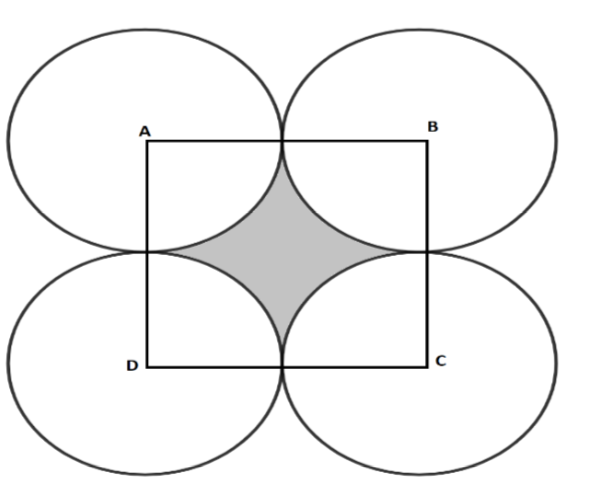Courses
Courses for Kids
Free study material
Free LIVE classes
MoreLIVE
Join Vedantu’s FREE Mastercalss

# In fig. ABCD is a square of side 14 cm, with centers A, B, C, D. Four circles are drawn such that each circle touches externally two of the remaining three circles. Find the area of the shaded region.Verified
363.3k+ views
Hint: Quadrant is one-fourth part of the circle and four quadrants sum up to become a circle. The area of the shaded portion can be found by subtracting the area of the square and the area of the four quadrants.

We know that the square makes a right angle at vertices. And it is given in the question that A, B, C and D are the centers of respective circles.
Considering the figure given in the questionWe need to find the area of the shaded region.
We know that the circle sector which makes a right angle at the center is known as a quadrant.
By looking at the figure we can see that the parts of each circle lying inside the square represent the quadrant of the respective circles.
Now, we need to find the radius of the circle. Let the radius of each circle be r.
Circles are of the same size with the same radius so, observing the given figure we can say that $2\times r=$ side of the square.
AB is the side of the square which is equal to 14 cm as given in the question.
\begin{align} & 2\times r=14 \\ & r=7cm \\ \end{align}
Now, we need to find the area of the shaded region given in the question.
Observing the figure,
Area of shaded region $=$ Area of square $-$ Area of the sum of 4 quadrants. …(1)
Now, we know that the area of the square $={{(side)}^{2}}$.
And, we also know that quadrant is one-fourth part of the circle and four quadrants sums to become a circle.
So, we can say that the area of the sum of 4 quadrants is equal to the area of a circle.
The area of the circle $=\pi {{r}^{2}}$.
Now, we can substitute the above result into equation (1).
Area of shaded region $=$ Area of square $-$ Area of the sum of 4 quadrants.
Area of the shaded region $=$${{(14)}^{2}}-\pi {{(7)}^{2}}$
Area of the shaded region $=196-\pi (49)$
Putting the value of $\pi =\dfrac{22}{7}$.
Area of shaded region $=42$ sq. Unit

Note: We can use an alternate method to find the individual area of the sector to find the area of the shaded region, as the area of the quadrant is given as $=\pi {{r}^{2}}\dfrac{{{360}^{\circ }}}{\theta }$ where $\theta$ is the angle made by sector at the center and we know that quadrant makes ${{90}^{\circ }}$ at the center so, the area of quadrant $=\dfrac{\pi {{r}^{2}}}{4}$.
Last updated date: 26th Sep 2023
Total views: 363.3k
Views today: 6.63k